# Critical line and critical strip

The critical line is the line of symmetry in the functional equation of the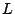-function. In the usual normalization the functional equation associates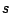to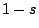, so the critical line is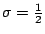.

In the usual normalization the Dirichlet series and the Euler product converge absolutely for The functional equation maps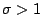to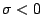. The remaining region,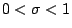is known as the critical strip.

By the Euler product there are no zeros in, and by the functional equation there are only trivial zeros in. So all of the nontrivial zeros are in the critical strip, and the Riemann Hypothesis asserts that the nontrivial zeros are actually on the critical line.

Back to the main index for The Riemann Hypothesis.